# Introduction to CaliCo

#### 2018-07-24

The package CaliCo allows to rule a Bayesian calibration on every kind of code (“black box” or analytic codes). The aim of the Bayesian calibration is to better asses the uncertainty on the parameters of the code. In CaliCo, the code to calibrate has to be defined with two kinds of inputs. The first concerns the forced variables and the second the parameters. f:\begin{align} \mathbb{R}^2 & \mapsto \mathbb{R} \\ (x,\theta) &\rightarrow y \end{align} If the code takes several forced variables and parameters, vectors can be used. f:\begin{align} \mathbb{R}^d\times\mathbb{R}^p & \mapsto \mathbb{R} \\ (\boldsymbol{x},\boldsymbol{\theta}) &\rightarrow y \end{align}

When design matrices are used (especially for the forced variables), the matrix can be implemented straightforwardly

f:\begin{align} \mathcal{M}_{n,d}\times\mathbb{R}^p & \mapsto \mathbb{R}^n \\ (\boldsymbol{X},\boldsymbol{\theta}) &\rightarrow \boldsymbol{y} \end{align}

Let us consider, for this introduction, a dynamic code with one parameter defined as f_t:\begin{align} \mathbb{R}^2 & \mapsto \mathbb{R} \\ (t,\theta) &\rightarrow (6t-2)^2sin(\theta t-4) \end{align}

The function $$f_t$$ is chosen with only one forced variable and one parameter $$\theta$$. Let us say that experiments are available and can be expressed by $$Y_exp$$. The belief on the value of $$\theta$$ shows that a non-negligible gap is present.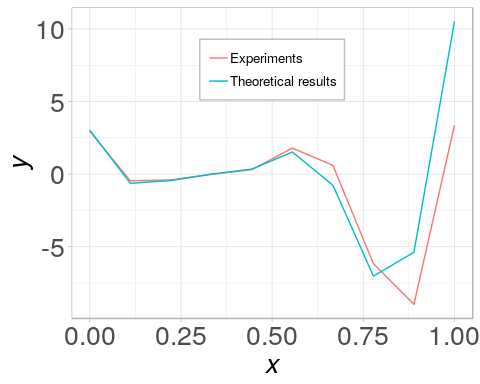The aim of calibration is to better asses the uncertainty on $$\theta$$ and access the variance of the output from the updated distribution. Several statistical model are available to realize such a study. The need to introduce four statistical models lies in the fact that one could encounter two major difficulities in code calibration. The first is when the code is time consuming and the second is when the code creates a discrepancy. That is why, the choice of the statistical model depends on each particular case (see Carmassi et al. 2018). The four statistical models implemented are detailed below.

The first model available is: $\mathcal{M}_1:\forall i \in [1,\dots,n] \ Y_{exp_i}=f(\boldsymbol{x_i},\boldsymbol{\theta})+\epsilon_i$ where $$Y_{exp_i}$$ stands for the $$i^{th}$$ from $$n$$ observations, $$\boldsymbol{x_i}$$ for the vector of controlled variables corresponding, and $$\epsilon_i$$ for the measurement error. In CaliCo, $$\epsilon$$ will always be defined as a white Gaussian noise with $$\epsilon \overset{iid}{\sim}\mathcal{N}(0,\sigma_{err}^2)$$. $$\sigma_{err}^2$$ stands for the variance of the measurement error and has to be found as much as $$\boldsymbol{\theta}$$.

The second model intervienes when the code is too long to run. In that case: $\mathcal{M}_2:\forall i \in [1,\dots,n] \ Y_{exp_i}=\boldsymbol{F}(\boldsymbol{x_i},\boldsymbol{\theta})+\epsilon_i$ where $$\boldsymbol{F}(\{\bullet,\bullet\})\sim\mathcal{PG}(m(\{\bullet,\bullet\}),c(\{\bullet,\bullet\},\{\bullet,\bullet\}))$$ is a Gaussian process defined for an expectancy $$m$$ and covariance $$c$$ functions.

The third model lies on $$\mathcal{M}_1$$ in the way that we consider another error term called discrepancy. $\mathcal{M}_3:\forall i \in [1,\dots,n] \ Y_{exp_i}=f(\boldsymbol{x_i},\boldsymbol{\theta})+\delta(\boldsymbol{x_i})+\epsilon_i$ where $$\delta(\boldsymbol{x_i})\sim\mathcal{PG}(m(\bullet),c(\bullet,\bullet))$$ is a Gaussian process quantifying the code error (or discrepancy).

In CaliCo, $$m(\bullet)$$ in discrepancy is set to zero for now.

Similarly, the fourth model is defined from $$\mathcal{M}_2$$ by adding the discrepancy: $\mathcal{M}_4:\forall i \in [1,\dots,n] \ Y_{exp_i}=F(\boldsymbol{x_i},\boldsymbol{\theta})+\delta(\boldsymbol{x_i})+\epsilon_i$

To run a Bayesian calibration in CaliCo, the statistical model must be chosen first. Then the prior densities of the parameters has to be set up as well. Then the calibration can be executed by the function calibrate.

## Define the statistical model

As detailed before, there is four models. Two of them use a Gaussian process to emulate the code. The users is free to control the parameters of the estimation which is realized by the DiceKrigging package (see (Roustant, Ginsbourger, and Devills 2012)).

Let us consider, the set of experiments generated for $$\theta=10.9$$ and a measurement error of $$\sigma_{err}^2=0.01$$. Let us also consider that the prior belief on $$\theta$$ is that $$\theta \sim \mathcal{N}(11,0.01)$$ and $$\sigma_{err}^2\sim\Gamma(1,0.01)$$.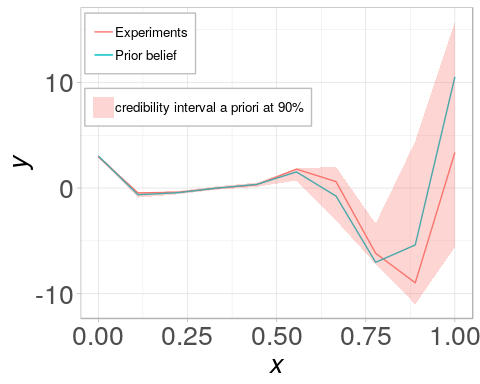After $$100$$ realizations of the prior densities, the credibility interval a priori at 90% is wide and it can be overly imprecise for one purpose.

### Model 1

To implement $$\mathcal{M}_1$$ in CaliCo, it is only necessary to:

library(CaliCo)
# Number of experiments
n=10
# Time interval
t <- seq(0,1,length.out=n)
# Code definition
code <- function(t,theta)
{
return((6*t-2)^2*sin(theta*t-4))
}
# Generate the experiment
Yexp <- code(t,10.5)+rnorm(n,0,sqrt(0.01))
# Generate the first model
model1 <- model(code=code,X=t,Yexp=Yexp,model="model1")

The function model creates a model.class object which is a R6 object. All the fields are accessible from the object created by the operator $ but two main methods are implemented, are print and plot, are usable as functions. print(model1) ## Call: ##  "model1" ## ## With the function: ## function(t,theta) ## { ## return((6*t-2)^2*sin(theta*t-4)) ## } ## ## No surrogate is selected ## ## No discrepancy is added To access graphical visualization of the model, parameter values need to be added. The pipe \%<\% defined is CaliCo allows to load parameter values into a statistical model. The argument of the pipe is a list which has for name theta, thetaD and var. These values stand for, respectively, the input parameter vector, the vector composed of the parameter of the discrepancy (only for $$\mathcal{M}_3$$ and $$\mathcal{M}_4$$, not necessary for $$\mathcal{M}_1$$ or $$\mathcal{M}_2$$) wich are the variance of the covariance function and the correlation lenght, and the variance of the measumrement error. The procedure for $$\mathcal{M}_1$$ is done as the following code line. model1 %<% list(theta=11,var=0.01) ## Warning: Please be carefull to the size of the parameter vector A Warning message appears everytime to sensitize the user to be careful with the size of the paramerter vector. Then to display the results of the model, the function plot can be used straightforwardly. The first argument of the function is the model, one wants to plot and the second argument is the x-axis. These two arguments are required to get the graph output. An additional argument, CI, allows to control the credibility interval one wants to see. By default CI="all", but if CI="err" only the $$95\%$$ CI of the measurment error with or not the discrepancy is given. Similarly, for $$\mathcal{M}_2$$ and $$\mathcal{M}_4$$ if CI="GP" only the $$95\%$$ CI of the Gaussian process is shown. The plot requires some inputs ( plot(model,theta,var)) where theta is the parameter value or vector and var the variance of the white Gaussian noise which represents the measurmement error. plot(model1,t)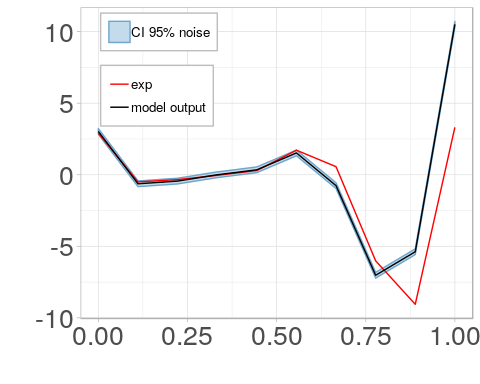Note that the method plot generates a ggplot. It is possible to store the plot into a variable and make some changes. For example, if one is interested in adding a title, the labels, decrease the size of the legend and move it a little: library(ggplot2) p <- plot(model1,t) p+ggtitle("Model1 and experiments")+ylab("y")+xlab("t")+ theme(legend.position=c(0.3,0.75),legend.text=element_text(size = '7'))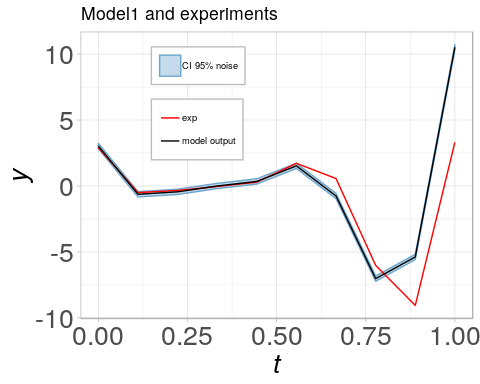### Model 2 The second model is usefull when the code is time consuming. The package CaliCo offers a flexibility from this point with three possibilities. The first, one possesses the code explicitely in R with the same features than before (note that if the code has no input variables, the function needs to be defined indentically as before but in the function model only set X=0) but without any design of experiments (DOE). In that cas, the function model creates a new maximin Latin Hypercube Space (LHS) and evaluates the code at the locations selected by the DOE. The options to establish such a DOE are controlled by the user with the option opt.emul. If one possesses the code and a specific DOE, it is also possible to give both of them at the function model. Then, if no code is available but a DOE with the corresponding outputs of the code are in the user’s possession, another option opt.sim allows to build the model on these specifications. Let us detail these three possibilities. #### Numerical code without DOE In this case, one possesses the code $$f_t$$ and has no DOE. To create a Gaussian process that emulates the code, an option needs to be filled in the function model which is opt.gp. This option is a list of two components: • type the type of the kernel in agreement with km function from DiceKrigging package • DOE specific DOE given by the user (default value NULL). If this case DOE is let to the NULL value. Then, the option opt.emul is useful to control the building of the DOE and is composed of: • p the number of parameter in the code • n.emul the number of experiments in the DOE (default value 100) • binf the lower bound of the parameter value or vector (default value 0) • bsup the upper bound of the parameter value or vector (default value 1) The creation of the model is completed by the following code. model2code <- model(code,X,Yexp,"model2", opt.gp=list(type="matern5_2", DOE=NULL), opt.emul=list(p=1,n.emul=40,binf=8,bsup=13)) ## ## optimisation start ## ------------------ ## * estimation method : MLE ## * optimisation method : BFGS ## * analytical gradient : used ## * trend model : ~1 ## * covariance model : ## - type : matern5_2 ## - nugget : NO ## - parameters lower bounds : 1e-10 1e-10 ## - parameters upper bounds : 1.96072 9.871572 ## - best initial criterion value(s) : -92.8202 ## ## N = 2, M = 5 machine precision = 2.22045e-16 ## At X0, 0 variables are exactly at the bounds ## At iterate 0 f= 92.82 |proj g|= 2.882 ## At iterate 1 f = 90.814 |proj g|= 0.90827 ## At iterate 2 f = 89.946 |proj g|= 0.24289 ## At iterate 3 f = 89.877 |proj g|= 1.7373 ## At iterate 4 f = 89.856 |proj g|= 0.4596 ## At iterate 5 f = 89.844 |proj g|= 0.22571 ## At iterate 6 f = 89.843 |proj g|= 0.13763 ## At iterate 7 f = 89.843 |proj g|= 0.013813 ## At iterate 8 f = 89.843 |proj g|= 6.8748e-05 ## ## iterations 8 ## function evaluations 11 ## segments explored during Cauchy searches 9 ## BFGS updates skipped 0 ## active bounds at final generalized Cauchy point 0 ## norm of the final projected gradient 6.8748e-05 ## final function value 89.8426 ## ## F = 89.8426 ## final value 89.842577 ## converged ##  "The surrogate has been set up, you can now use the function" The output is the results of the optimization done by DiceKriging for estimating the parameters of the Gaussian process. #### Numerical code with DOE In that case, one is free to generate a DOE appart from the function model. Let us consider, for the following, that the DOE is generated by the package DiceDesign, is a maximin LHS of $$30$$ points and is called myDOE. Then, to built the model, the option opt.emul is not needed anymore. model2doe <- model(code,X,Yexp,"model2", opt.gp=list(type="matern5_2", DOE=myDOE)) ## ## optimisation start ## ------------------ ## * estimation method : MLE ## * optimisation method : BFGS ## * analytical gradient : used ## * trend model : ~1 ## * covariance model : ## - type : matern5_2 ## - nugget : NO ## - parameters lower bounds : 1e-10 1e-10 ## - parameters upper bounds : 1.912401 9.842393 ## - best initial criterion value(s) : -74.58473 ## ## N = 2, M = 5 machine precision = 2.22045e-16 ## At X0, 0 variables are exactly at the bounds ## At iterate 0 f= 74.585 |proj g|= 2.4205 ## At iterate 1 f = 73.711 |proj g|= 2.5748 ## At iterate 2 f = 71.805 |proj g|= 2.2469 ## At iterate 3 f = 71.283 |proj g|= 1.7387 ## At iterate 4 f = 71.09 |proj g|= 0.21966 ## At iterate 5 f = 70.99 |proj g|= 0.20329 ## At iterate 6 f = 70.989 |proj g|= 0.032853 ## At iterate 7 f = 70.989 |proj g|= 0.018525 ## At iterate 8 f = 70.989 |proj g|= 0.0020157 ## At iterate 9 f = 70.989 |proj g|= 9.2632e-06 ## ## iterations 9 ## function evaluations 13 ## segments explored during Cauchy searches 10 ## BFGS updates skipped 0 ## active bounds at final generalized Cauchy point 0 ## norm of the final projected gradient 9.2632e-06 ## final function value 70.9886 ## ## F = 70.9886 ## final value 70.988640 ## converged ##  "The surrogate has been set up, you can now use the function" #### DOE with corresponding outputs In this case, an option is added to opt.gp and allows define a DOE and the corresponding outputs of the code. This option opt.sim is a list of two components: • Ysim the outputs of the code • DOEsim the DOE corresponding Let us consider that for myDOE all the points are evaluated by $$f_t$$ and the outputs are collected into a vector called Ys. Then, the model definition is completed with the following code: model2 <- model(code=NULL,X,Yexp,"model2", opt.gp=list(type="matern5_2", DOE=NULL), opt.sim=list(Ysim=Ys,DOEsim=myDOE)) ## ## optimisation start ## ------------------ ## * estimation method : MLE ## * optimisation method : BFGS ## * analytical gradient : used ## * trend model : ~1 ## * covariance model : ## - type : matern5_2 ## - nugget : NO ## - parameters lower bounds : 1e-10 1e-10 ## - parameters upper bounds : 1.912401 9.842393 ## - best initial criterion value(s) : -81.47316 ## ## N = 2, M = 5 machine precision = 2.22045e-16 ## At X0, 0 variables are exactly at the bounds ## At iterate 0 f= 81.473 |proj g|= 1.8369 ## At iterate 1 f = 81.179 |proj g|= 2.0426 ## At iterate 2 f = 80.545 |proj g|= 2.3932 ## At iterate 3 f = 78.92 |proj g|= 3.1905 ## At iterate 4 f = 76.165 |proj g|= 2.5504 ## At iterate 5 f = 74.421 |proj g|= 2.1475 ## At iterate 6 f = 71.603 |proj g|= 2.0668 ## At iterate 7 f = 71.024 |proj g|= 1.5005 ## At iterate 8 f = 71 |proj g|= 0.38531 ## At iterate 9 f = 70.989 |proj g|= 0.065934 ## At iterate 10 f = 70.989 |proj g|= 0.016629 ## At iterate 11 f = 70.989 |proj g|= 0.00095408 ## At iterate 12 f = 70.989 |proj g|= 3.1266e-06 ## ## iterations 12 ## function evaluations 18 ## segments explored during Cauchy searches 13 ## BFGS updates skipped 0 ## active bounds at final generalized Cauchy point 0 ## norm of the final projected gradient 3.12658e-06 ## final function value 70.9886 ## ## F = 70.9886 ## final value 70.988640 ## converged ##  "The surrogate has been set up, you can now use the function" #### Graphical representation Similarly as for $$\mathcal{M}_1$$, parameters has to be added with the pipe \%<\% to access visual representation. paramList <- list(theta=11,var=0.1) model2code %<% paramList ## Warning: Please be carefull to the size of the parameter vector model2doe %<% paramList ## Warning: Please be carefull to the size of the parameter vector model2 %<% paramList ## Warning: Please be carefull to the size of the parameter vector plot(model2code,t)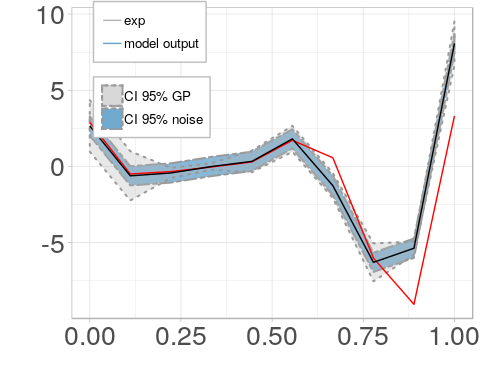plot(model2doe,t)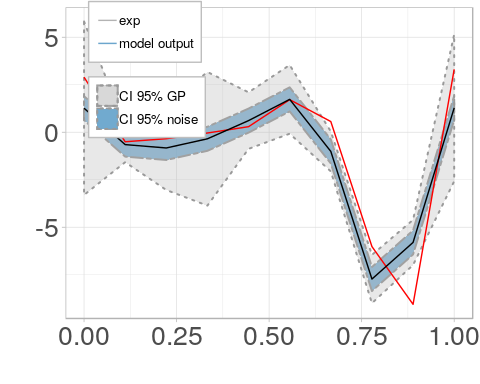plot(model2,t)### Model 3 and Model 4 Both $$\mathcal{M}_3$$ and $$\mathcal{M}_4$$ add a discrepancy to respectively $$\mathcal{M}_1$$ and $$\mathcal{M}_2$$. Then discrepancy options has to be filled in the model definition. This option is called opt.disc and is a list of only one element kernel.type (further development will be brought in the future to better control the discrepancy by adding an expectancy form). The kernel.type can be: • “gauss” Gaussian • “exp” Exponential • “matern3_2” Matèrn 3/2 • “matern5_2” Matèrn 5/2 The user is free to select the form of the covariance structure in the discrepancy. Howerver, the expectancy is set automatically to zero. As an example, for $$\mathcal{M}_3$$ no surrogate of the code is used then: model3 <- model(code,X,Yexp,"model3", opt.disc=list(kernel.type="matern5_2")) For $$\mathcal{M}_4$$, in the third case (where only the DOE and code output are available) the model is generated by: model4 <- model(code,X,Yexp,"model4", opt.gp=list(type="matern5_2", DOE=NULL), opt.sim=list(Ysim=Ys,DOEsim=myDOE), opt.disc=list(kernel.type="gauss")) ## ## optimisation start ## ------------------ ## * estimation method : MLE ## * optimisation method : BFGS ## * analytical gradient : used ## * trend model : ~1 ## * covariance model : ## - type : matern5_2 ## - nugget : NO ## - parameters lower bounds : 1e-10 1e-10 ## - parameters upper bounds : 1.912401 9.842393 ## - best initial criterion value(s) : -72.11872 ## ## N = 2, M = 5 machine precision = 2.22045e-16 ## At X0, 0 variables are exactly at the bounds ## At iterate 0 f= 72.119 |proj g|= 2.2831 ## At iterate 1 f = 71.295 |proj g|= 1.2415 ## At iterate 2 f = 71.265 |proj g|= 1.3837 ## At iterate 3 f = 71.252 |proj g|= 1.4252 ## At iterate 4 f = 71.222 |proj g|= 1.7046 ## At iterate 5 f = 71.155 |proj g|= 1.7122 ## At iterate 6 f = 71.057 |proj g|= 1.7183 ## At iterate 7 f = 70.998 |proj g|= 1.7162 ## At iterate 8 f = 70.989 |proj g|= 0.71956 ## At iterate 9 f = 70.989 |proj g|= 0.02065 ## At iterate 10 f = 70.989 |proj g|= 0.00078269 ## At iterate 11 f = 70.989 |proj g|= 2.1703e-06 ## ## iterations 11 ## function evaluations 14 ## segments explored during Cauchy searches 12 ## BFGS updates skipped 0 ## active bounds at final generalized Cauchy point 0 ## norm of the final projected gradient 2.17027e-06 ## final function value 70.9886 ## ## F = 70.9886 ## final value 70.988640 ## converged ##  "The surrogate has been set up, you can now use the function" Identically as before, parameters are added. However, the parameters relative to the discrepancy needs to be added. As a matter of fact, these parameters are estimated in Bayesian calibration at the same time than theta and var. That is why a parameter vector thetaD is added and contains $$\sigma_{\delta}^2$$ and $$\psi$$ the variance and the correlation length of the Gaussian process of the discrepancy. model3 %<% list(theta=11,thetaD=c(0.1,0.5),var=0.1) ## Warning: Please be carefull to the size of the parameter vector model4 %<% list(theta=11,thetaD=c(0.1,0.5),var=0.1) ## Warning: Please be carefull to the size of the parameter vector plot(model3,t)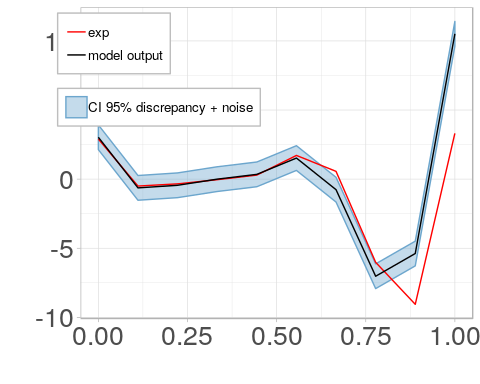plot(model4,t)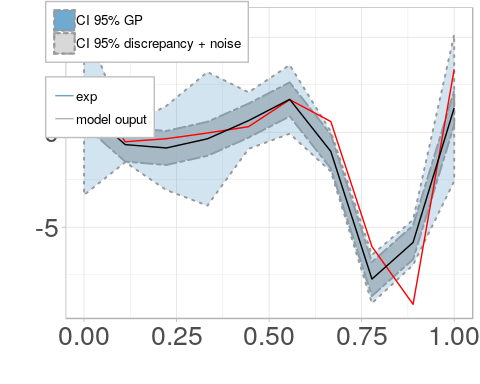## Define the prior distributions In Bayesian calibration a prior distribution is updated in a posterior distribution thanks to the likelihood. The prior distribution, in CaliCo, is defined as the following chunk. gaussian <- prior(type.prior="gaussian",opt.prior=list(c(0.5,0.001))) plot(gaussian)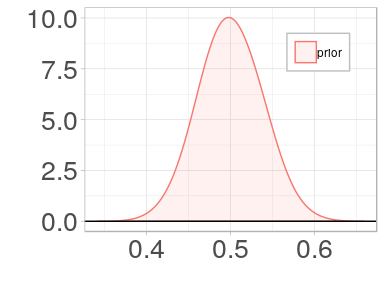For calibration with $$\mathcal{M}_1$$ and $$\mathcal{M}_2$$, only theta and var are calibrated. That means in code calibration of $$f_t$$ only two prior densities are defined. To define multiple prior densities in CaliCo, one can create a vector of characters for the option type.pior and a list containing the corresponding vectors of the hyperparameters of the distribution. Then in the example: pr1 <- prior(type.prior=c("gaussian","gamma"), opt.prior=list(c(11,0.01),c(1,0.1))) plot(pr1$Prior1)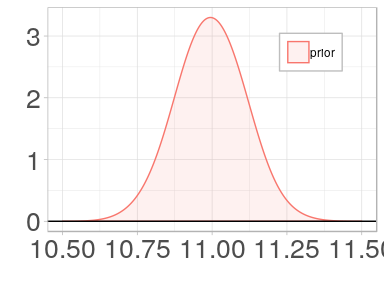plot(pr1$Prior2)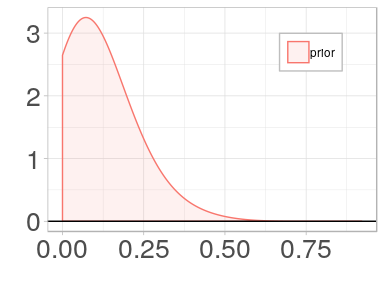The function prior in this particular case creates a list containing both prior densities. If one is interrested in visualizing the densities, the function plot, used as in the previous code chunck, allow to access the ggplot2 object directly. The list contains prior density in the order set in the prior function. That means for the example given above, Prior1 corresponds to the Gaussian density and Prior2 to the Gamma density (respectively to theta density and var density). For $$\mathcal{M}_3$$ and $$\mathcal{M}_4$$, two additionnal parameters are needed before running calibration. One can define them identically as before. pr2 <- prior(type.prior=c("gaussian","gamma","unif","gamma"), opt.prior=list(c(11,0.01),c(1,2),c(0,1),c(1,0.1))) plot(pr2$Prior2)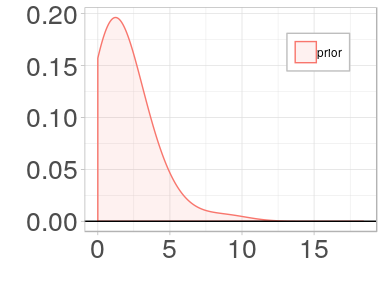plot(pr2$Prior3)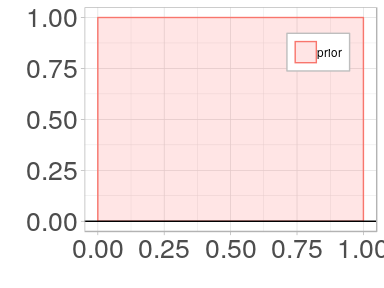In CaliCo the order is always defined by theta first, then if there is a discrepancy thetaD and then the variance of the measurment errors var. ## Run Bayesian calibration Let us recall that if $$\mathcal{M}_1$$ or $$\mathcal{M}_2$$ are selected only $$\theta$$ and $$\sigma_{err}^2$$ need to be calibrated. If $$\mathcal{M}_3$$ or $$\mathcal{M}_4$$ are chosen $$\theta$$, $$\theta_{\delta}$$ and $$\sigma_{err}^2$$ need to be calibrated. In CaliCo, estimation options have to be defined to run the calibration (opt.estim). It encompasses a list of the options to run the Monte Carlo Markov Chain (MCMC): • Ngibbs the number of Gibbs in the Metropolis within Gibbs algorithm • Nmh the number of Metropolis Hastings in the Metropolis within Gibbs algorithm • thetaInit the starting point • r the tuning vector of k which regulate the covariance matrix in the proposition distribution($$k\Sigma$$). Each $$100$$ iteration in the MCMC, $$k = k(1-r)$$ is realized if the acceptation rate is lower than $$20\%$$ and $$k = k(1+r)$$ if the acceptation rate is higher than $$50\%$$. • sig the covariance matrix in the symetric proposition distribution • Nchains the number of MCMC chains (if Nchains>1 an output called mcmc is a coda object and can be manipulated to run a Gelman Rubin diagnostic for example) • burnIn the burn-in to take of the final sample set Between $$\mathcal{M}_1$$, $$\mathcal{M}_2$$ and $$\mathcal{M}_3$$ and $$\mathcal{M}_4$$, opt.estim is not the same because of the size of thetaInit and sig. Indeed, with the discrepancy, the number of parameter to calibrate had increased of two. The parameter r can remain the same between the models. The function calibrate is the function that run the Bayesian calibration. It takes three inputs: • md the model.class object generated by the function model • pr the prior.class object generated by the function prior • opt.estim the estimation options mdfit1 <- calibrate(model1,pr1, opt.estim = list(Ngibbs=200,Nmh=400,thetaInit=c(11,0.01), r=c(0.3,0.3),sig=diag(2),Nchains=1,burnIn=100)) One can easily access to a summary of the results by running a print(mdfit1). print(mdfit1) ## Call: ## ## With the function: ## function(t,theta) ## { ## return((6*t-2)^2*sin(theta*t-4)) ## } ## <bytecode: 0x55813e44cae0> ## ## Selected model : model1 ## ## Acceptation rate of the Metropolis within Gibbs algorithm: ##  "84%" "79.5%" ## ## Acceptation rate of the Metropolis Hastings algorithm: ##  "60.75%" ## ## Maximum a posteriori: ##  10.51820734 0.02585177 ## ## Mean a posteriori: ##  10.51048141 0.01661772 The summary recalls the function used, the selected model but indicates the rates of the algorithms. Then, the Maximum A Posteriori (MAP) and the mean a posteriori are also plotted. One can also have access to a visual representation of the results proposed by CaliCo. With the plot function, one can display a serie of graphs. Similarly, as the plot function used to display the model, the second argument is required and is the x-axis. plot(mdfit1,t) ## Warning: No correlation plot available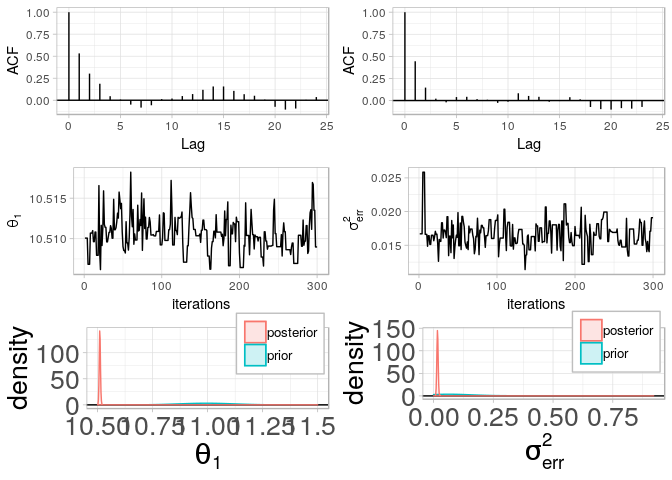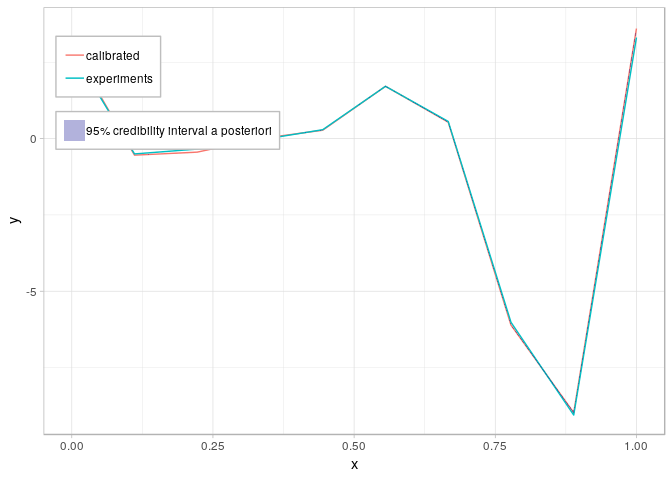With only one parameter to calibrate in theta, the Warning indicates that no correlation graph is available. As a matter of fact, a second group of graphs in given with the previous ones which is correlation plot between each parameters theta with the corresponding densities a priori and a posteriori. One can control this output with an additionnal option graph: • if graph="chains": only the table of the autocorrelation, chains points and densities a priori and a posteriori is produced. • if graph="corr": only the table of the correlation graph between each parameter is displayed. • if graph="result": only the result on the quantity of interest is given.. • if graph=NULL: no graphs are produced automatically. The serie of graphs is just a display proposition by CaliCo. One can elect the specific graph he want to plot. All the graph are ggplot2 objects and are generated in a list. For example, if one runs p <- plot(mdfit1,t), p is a list containing several elements: • ACF a list of all autocorrelation graphs in the chains for each variable • MCMC a list of all the MCMC chains for each variable • corrplot a list of all correlation graphs between each variable • dens a list of all density a priori and a posteriori graphs for each variable • out the result on the quantity of interest These list of graphs are also only propositions. One is free to load the generated MCMC chain (without the burn-in sample) and produces the graphical representation he wants. This operation is done by the function chain: mcmc <- chain(mdfit1) head(mcmc) ## Markov Chain Monte Carlo (MCMC) output: ## Start = 1 ## End = 7 ## Thinning interval = 1 ## [,1] [,2] ## [1,] 11.00000 0.01000000 ## [2,] 10.51314 0.01424149 ## [3,] 10.51407 0.01378605 ## [4,] 10.51407 0.01378605 ## [5,] 10.51308 0.01680824 ## [6,] 10.51396 0.01614237 ## [7,] 10.51079 0.01699770 To also quickly get the estimators of the chain, the function estimators return the mean a posteriori and the MAP: Estimators <- estimators(mdfit1) print(Estimators) ##$MAP
##  10.5105471  0.0164484
##
## \$MEAN
##  10.51048141  0.01661772

The calibration procedure is identical for $$\mathcal{M}_2$$. However, for $$\mathcal{M}_3$$ and $$\mathcal{M}_4$$ more parameters are to calibrate. That means the option opt.estim needs to be adapted. For example, in $$\mathcal{M}_4$$:

mdfit4 <- calibrate(model4,pr2,
opt.estim = list(Ngibbs=1000,Nmh=3000,thetaInit=c(11,2,0.5,0.01),
r=c(0.3,0.3),sig=diag(4),Nchains=1,burnIn=1000))

The computing time for calibrating $$\mathcal{M}_3$$ and $$\mathcal{M}_4$$ is much longer than calibrating $$\mathcal{M}_1$$ and $$\mathcal{M}_2$$. The matrix inversion in the model’s likelihood is reponsible of the increase of time. Indeed, from $$\mathcal{M}_1$$ to $$\mathcal{M}_4$$ the complexity of the covariance matrix in the likelihood increases.

From this point, when calibration is done, all the functions used above: estimators, chain, plotand print are accessible.

## Run Bayesian forecasting

CaliCo also allows the user to access a prediction on a new data set regarding the already run calibration. In the example, let us consider that one is interested in visualizing what happen on $$$1,1.5$$$ interval. The function forecast simply gets two inputs which are the calibrated object mdfit and the new data set. In the example if x.new = seq(1,1.5,length.out=10) then the argument in the function forecast is x=x.new.

x.new <- seq(1,1.5,length.out=10)
fr <- forecast(mdfit1,x.new)

The function plot gives a visual representation on the predicted values for the chosen statistical model. The x-axis given has to be the original x-axis used for calibration extended with the x-axis corresponding to the new data set.

plot(fr,c(t,x.new))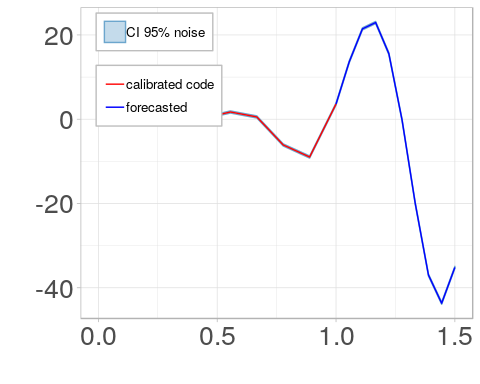The blue line corresponds to the predicted values and the credibility intervals at $$95\%$$ are also given depending on the chosen model.

## Conclusion

This vignette illustrates the use of CaliCo in a simple example. Although, for a multidimensional test, the reader can try out the examples given in the help ?model of ?calibrate. The aim of the package is to be adaptive to each industrial situation and makes Bayesian calibration accessible.